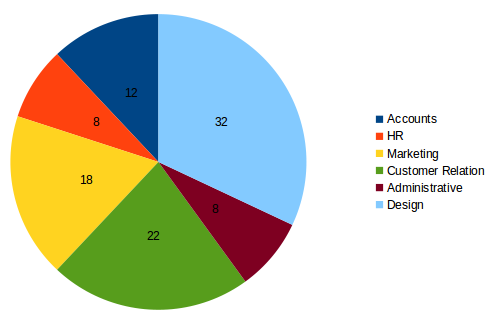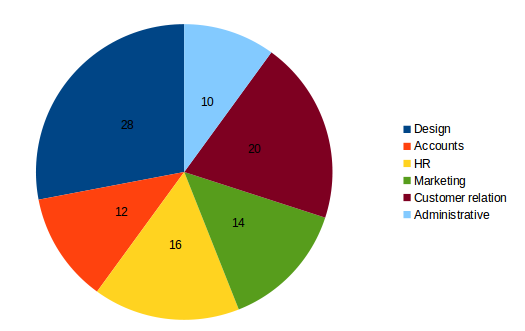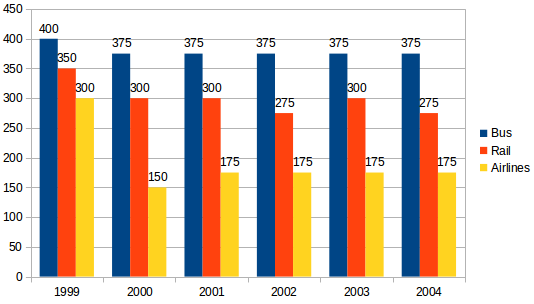## Data Interpretation For SBI PO : Set – 52

D.1-5) Study the following graph carefully and answer the questions that follow.

Percentage of employees in different departments of a company

Total No.of employees = 4500Percentage of females in each department in the same company

Total No of females in the organisation = 20001) What is the total number of males from Design, Customer Relation, and HR departments together?

a) 1550

b) 1510

c) 1540

d) 1580

e) None of these

b) 1510

b) Number if employees in design, customer relation and HR departments together

4500*(32+22+8)%=(4500*62)/100=2790

Number of women employees in these departments = 2000 * (28+20+16)%

=(2000*64)/100=1280

Required number of males = 2790-1280 = 1510

2) What is the ratio of number of males in HR department in HR department to the number of males in Accounts department respectively?

a) 3:17

b) 4:15

c) 2:15

d) 2:13

e) None of these

c) 2:15

Number of employees in HR department = (4500*8)/100=360

Number of males = 360-(2000*16)/100=360-320=40

Number of employees in Account department = (4500*12)/100=540

Number of males= 540-(2000*12)/100=540-240=300
Required Ratio = 40:300 = 2:15

3) The number of females in the Marketing department are approximately what percent of the total employee in Marketing and Customer Relation departments together?

a) 26

b) 36

c) 6

d) 46

e) 16

e) 16

Number of employees in marketing customer relation departments

= (4500*40)/100=1800

Number of females in the marketing department = (2000*14)/100=280

Required Percentage = (280/1800)*100=16

4) What is the respective ratio of number of employees in Administrative department to the number of males in the Same department?

a) 9:4

b) 8:3

c) 7:2

d) 8:5

e) None of these

a) 9:4

Total number of employees in administrative department =(4500*8)/100=360

Number of males in the Same department = 360-200=160

Required Ratio = 360:160=9:4

5) The total number of females are what percent of the total number of males in the organisation?

a) 90

b) 70

c) 80

d) 60

e) None of these

c) 80

Required Percentage = (2000/2500)*100=80

D.6-10) Study the following graph and answer the questions

Preferences of People in Using different Modes of Transport over the years6) From 1999 to 2004 the total number of people who preferred to travel by rail, was approximately how many millions?

a) 1300

b) 1500

c) 1600

d) 1800

e) 2000

d)

Total number of people travelling by rail =(350+300+300+275+300+275)millions

= 1800 millions

7) The number of people preferring to travel by rail in 2004, was how many millions fewer than the number of people preferring to travel by rail in 1999?

a) 70

b) 45

c) 75

d) 50

e) None of these

c)

Required difference = 350-275= 75 millions

8) In 2001, the people preferring to travel by bus represented approximately what per cent of the people preferring to travel by buses,rail and airlines together in that year?

a) 65 percent

b) 35 percent

c) 55 percent

d) 75 percent

e) 45 percent

e)

Total number of people travelling by buses,rail and airlines in 2001

= 375+300+175=850 millions

Number if people travelling by buses = 375millions

Required Percentage = (375/850)*100=45

9) What is the respective ratio of the number of people preferring to travel by bus to the number of people preferring to travel by rail in the year 2002?

a) 15:11

b) 9:7

c) 7:9

d) 11:15

e) None of these

a)

Required ratio = 375:275 = 15:11

10) In the year 2003 , if all the airlines reduced their rates by 50% and 50% of the people who preferred to travel by rail , now preferred airlines approximately how many millions of people prefer to travel by air?

a) 290

b) 313

c) 330

d) 300

e) 325